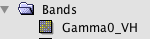# Calculate Backscatter coefficient by SNAP

Hi, I would like to estimate soil moisture from sentinel-1 GRD data.
So, I used SNAP for calibration sigma0 with several steps.

1. Sigma0 = backscatter coefficient ??(plz steps calibration)
2. how can I estimate soilmoisture from backscatter coefficient?

Thank you so much.

Please have a look at these two topics:

I want to know, Calibration in SNAP Data (Sigma0) = backscatter coefficient
Is this the end? Is there anything more I have to do for backscatter coefficient?

If you have strong topography, you can consider applying Terrain Flattening as described here: S1 radiometric correction

1. Orbit File
2. Calibrate to Sigma0
3. Speckle Filter
4. Terrain Correction
5. Convert to dB

I’ll use this step for backscatter coefficient.
You mean Num 5 is backscatter coefficient, right?

no, Sigma0 (Step 2) is the normalized radar cross section, or in short the backscatter coefficient. Conversion to dB scale is optional.

Then… you mean I can’t use that backsactter coefficient from sigma0?
But I know many published approaches use regressions to estimate soil moisture from sigma0…

I never said that - use itSo how can I derive the backscattering coefficient from S1A_IW_GRD products? I need backscattering coefficients to match my scattering model of electromagnetic wave. Consequently, exact backscattering coefficients are really important. Thank you, sir.

By using the radiometric calibration module

Thanks, sir. Here are my procedures:

3. Radar->Calibrate->(Processing Parameters: Output Beta0 band);

Is there anything wrong? Moreover, the result of pixel value after Step 5 is the EXACT backscattering coefficient? Thank you!

BTW, my data is S1A_IW_GRD…

I see nothing wrong with that.
You will still have some radiometric inaccuracies, maybe resulting from topography and the quality of the DEM in the terrain flattening step.

Excuse me, sir. What can I do more to obtain the exact backscattering coefficients? And, in my Step 3, the output result is Beta0 band. Should I output the Sigma0 band? What is the difference among Beta0, Sigma0 and Gamma0 band?

the only thing I can think of is to use digital elevation models of higher spatial resolution and quality.

Regarding your second question, you need Beta0 as an input for Terrain Flattening - please have a look here:

After Step 5, the output band is “Gamma0_VV” or “Gamma0_VH”?

VV and VH are different polarizations of the microwave, they are not related to the calibration process.

Yes. I wonder after Step 5, the output band is “Gamma0”?

This is the result after Step 5:Terrain Flattening converts Beta0 to Gamma0